Signed Distance Function (SDF): Implicit curves or surfaces

A mathematical equation usually involves the relationship between several unknowns. For exemple: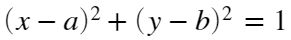In this case, the relation between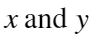is implicit because none of then are expressed in terms of the other. In case we clear one of the unknowns in terms of the other, this become an explicit equation for this unknown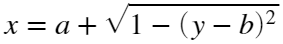2D SDF: Distance to a given point

When you consider an implicit equation and you equals it to zero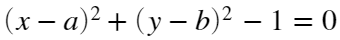the set of points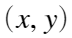that fulfill this equation defines a curve in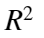(a surface in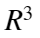). In our equation it corresponds to the set of points at distance 1 of the point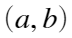, that is, a circle. This is the implicit curve defined by our equation, but we can also equals the implicit equation to a value d.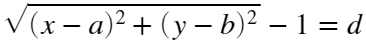Then, this is another implicit curve, now at a distance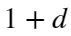of the point. Or what is the same, the set of points at a distance d of the circle.
For simplicity, let's consider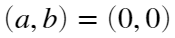the origin of coordinates and the signed distance function defined for all points inby: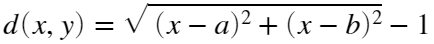If we evaluate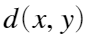in a grid of points defined in a chosen domain, we have (see the figure):
•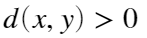The point is outside of the circle
•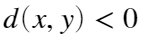The point is inside of the circle
•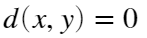The point is on of the circle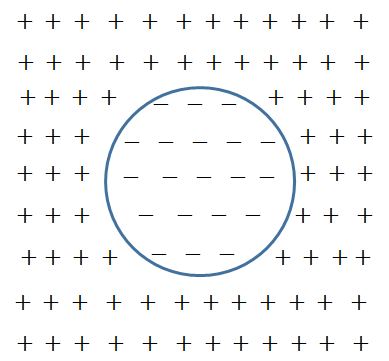The points on the unity cercle fulfill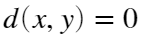x = -1.5:0.1:1.5;
y = -1.5:0.1:1.5;
[X,Y]=meshgrid(x,y);
d=sqrt(X.^2+Y.^2)-1;
dValues = [0,0]; %range of values of d to be plotted (only d=0 in this case)
figure
contour(X,Y,d,dValues)
grid on
axis equal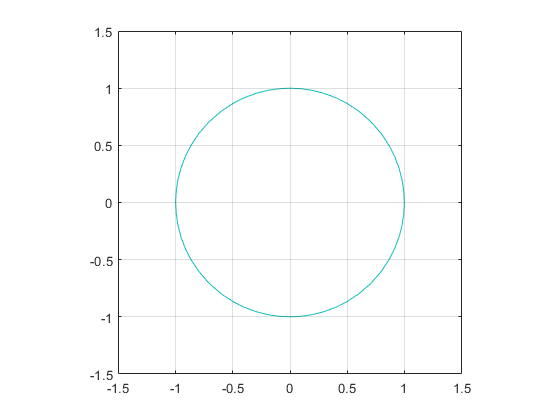dValues = [-0.2,0,0.3]; %range of values of d to be plotted (three curves)
figure
contour(X,Y,d,dValues,'ShowText','on') %show level curve
grid on
axis equal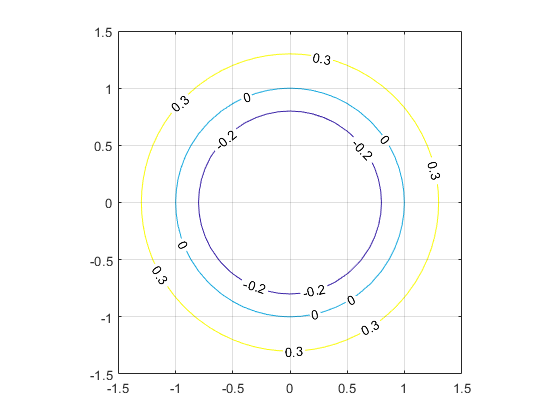Signed Distance Function 2D: Distance to a segment

In the case of a segment we have to consider the distance according of where the pointwe are measuring with respect to the segment is. In fact we have to compute which is the closest point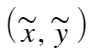in the segment and compute the distance between the two points.
Let's assume the segment is defined between two points A and B, then we can parametrize the segment as the set of points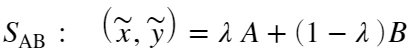If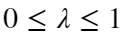the point belongs to the segment and for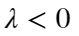or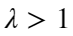you are in the same line but outside the segment (see the figure)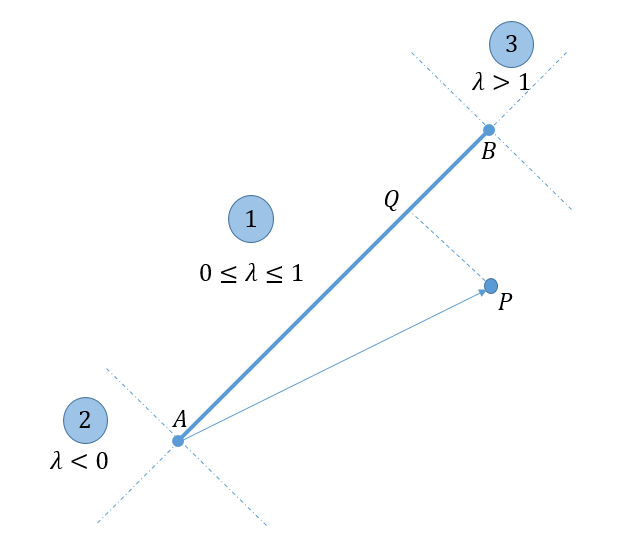In order to compute the value of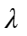, we only need to project the vector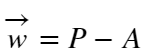to the line defined by point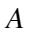and direction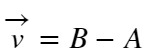.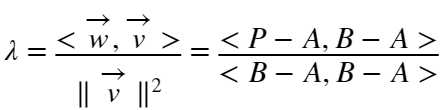and from this value, the closest point is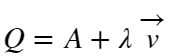(here <, > means dot product).
According to the value of, you will be in region 1, 2 or 3 (see figure) of the plane. Thus, we can have
•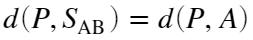if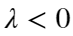(region 2)
•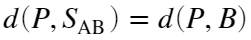if(region 3)
•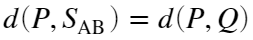if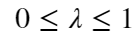(region 1)
You can code this in Matlab using some if conditions or you can obtain a compactify version of the formula according to that:
1. Because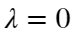correspond to point A, the distance whenis the same as the distance for, so we can clamp the values of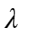using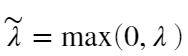2. The same argument can be applied to point B now with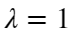and the values. So we can clamp now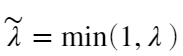3. Combining the previous comments, we can have only one final formula for our computation: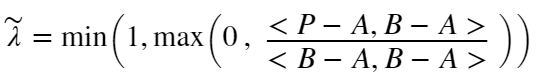. Then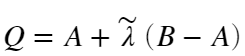andA = [0,0];
B = [2,2];
x = -1:0.1:3;
y = -1:0.1:3;
[X,Y] = meshgrid(x,y);
BmA = B-A;
den = BmA*BmA';
d = zeros(size(X));
for i = 1:numel(X)
P = [X(i),Y(i)];
lamb = dot(P-A, BmA)/den;
lambt = min(1, max(0, lamb));
Q = A+lambt*BmA;
d(i) = norm(P-Q);
end
dValues = [0, 0.1, 0.2, 0.3, 0.4]; %range of values of d to be plotted (d=0 in this case)
figure
contour(X,Y,d,dValues)
grid on
axis equal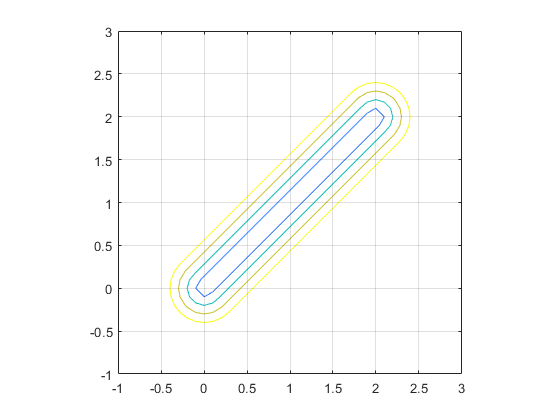Signed Distance Function 3D: Distance to a segment

The same formulation of the case 2D can be implemented in 3D. In fact, all the formulas are vectorial formulas and are independent of the number of coordinates. You only need to consider a third coordinate for all the points, the procedure is exactly the same.
In Matlab the function to get the contours is named isosurface. Below you can see how to use it.
A = [0,0,0];
B = [2,2,2];
x = -1:0.05:3;
y = -1:0.05:3;
z = -1:0.05:3;
[X,Y,Z] = meshgrid(x,y,z);
BmA = B-A;
den = BmA*BmA';
d = zeros(size(X));
for i = 1:numel(X)
P = [X(i),Y(i),Z(i)];
lamb = dot(P-A, BmA)/den;
lambt = min(1, max(0, lamb));
Q = A+lambt*BmA;
d(i) = norm(P-Q);
end
dVal=0.5;
figure
isosurface(X,Y,Z,d,dVal);
axis equal
view([28.36 -33.00])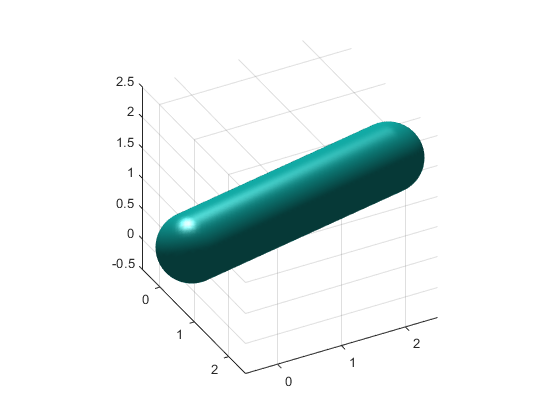Signed Distance Function 3D: Distance to a Box

This problem is similar to the distance of a segment but here we have to take into account symmetries and two segment. We plot in the figure the 2D case and implement directly the 3D one.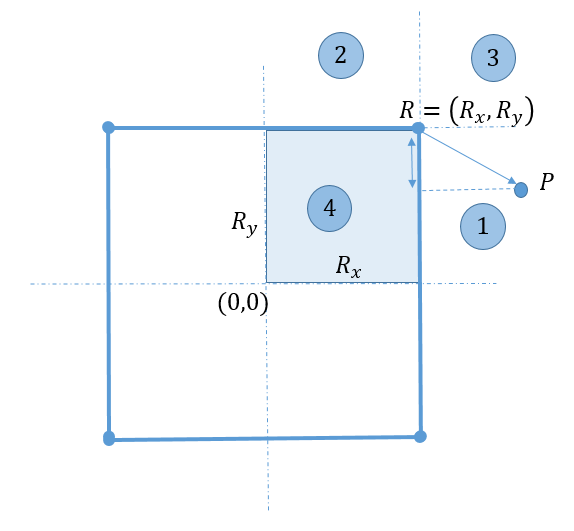Here we have
•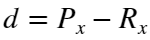for points in region 1
•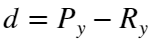for points in region 3
•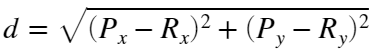for points in region 1
The formula to unify regions 1, 2 3 can be expressed as:
•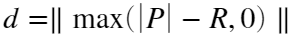, where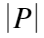means the absolute value of its coordinates.
The general formula can be derived to include also internal points in region 4 and is expressed as: (this is not as intuitive as the segment example)
•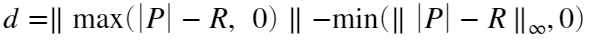where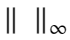is defined as the absolut value of the maximum component of a vector.
We can apply this formulas directly to 3D vectors
% Centered 3D Box
Rx=0.5;
Ry=0.5;
Rz=0.5;
R=[Rx,Ry,Rz];
x = -2:0.05:2;
y = -2:0.05:2;
z = -2:0.05:2;
[X,Y,Z] = meshgrid(x,y,z);
d = zeros(size(X));
for i = 1:numel(X)
P = [X(i),Y(i),Z(i)];
Q = abs(P)-R;
d(i) = norm(max(Q-R,0))-min(norm(abs(P)-R,"inf"),0);
end
dVal=0;
figure
isosurface(X,Y,Z,d,dVal);
axis equal
view([61.72 21.60])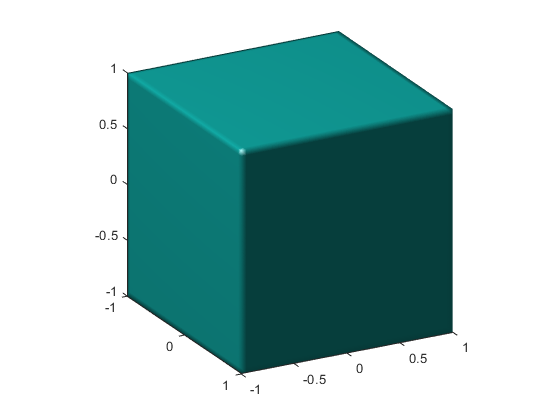dVal = 0.2; % now plot a little bit out of the zero surface
figure
isosurface(X,Y,Z,d,dVal);
axis equal
view([61.72 21.60])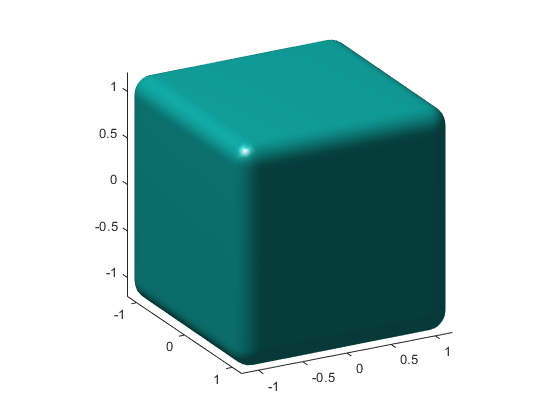Mixture of several objects

We now consider plotting the surface corresponding to many objects. When using signed distance functions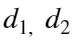to two different bodies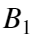and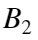the following properties apply (see the figure):
•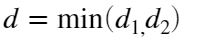gives the union of the two bodies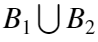•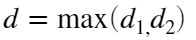gives the intersection of the two bodies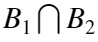•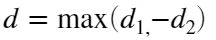gives the difference of the two bodies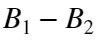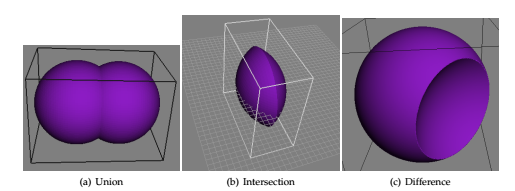Below we show an example using spheres and segments.

First sphere:

Let's now consider the distance to a point in 3D (in fact we consider square distance), that is a sphere. Like in the 2D case we can compute. We also introduce some lighting properties in order to obtain better visualizations.
% here we define the implicit function directly
x = -3:0.1:3;
y=x;
z=x;
[X,Y,Z]=meshgrid(x,y,z);
c = [0,0,0.5]; %center of the sphere
d1 = zeros(size(X));
for i=1:numel(X)
p=[X(i),Y(i),Z(i)];
d1(i)=(p(1)-c(1)).^2 +(p(2)-c(2)).^2 +(p(3)-c(3)).^2 - r^2;
end
dVal=0.0;
figure
patch(isosurface(X,Y,Z,d1,dVal,X),...
'FaceColor','interp','EdgeColor','none')
view(30,-15)
axis equal
grid
colormap gray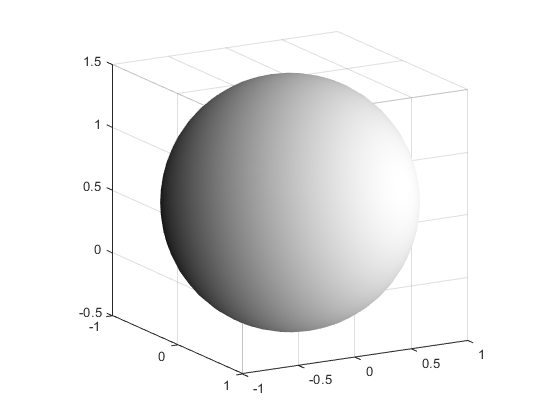Second sphere:

Now we add a second small sphere and we consider the union of the two spheres using the new distance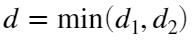% Add a new small sphere
c = [0,0,-0.5]; %center of the sphere
d2 = zeros(size(X));
for i=1:numel(X)
p=[X(i),Y(i),Z(i)];
d2(i)=(p(1)-c(1)).^2 +(p(2)-c(2)).^2 +(p(3)-c(3)).^2 - r^2;
end
dd=min(d1,d2);
dVal=0.2;
figure
patch(isosurface(X,Y,Z,dd,dVal,X),...
'FaceColor','interp','EdgeColor','none')
view(30,-15)
axis equal
grid
colormap gray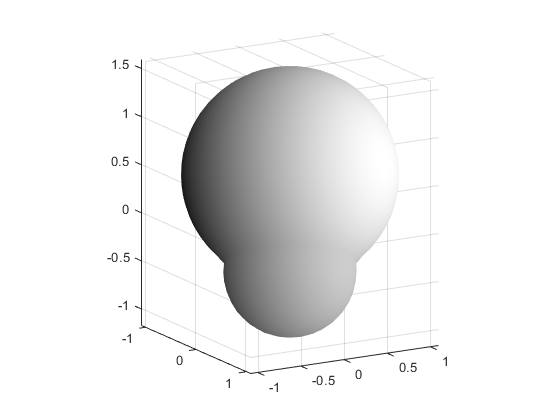Two Spheres and a Segment :

Finally we add a third object from the distance to a given segment using the new distance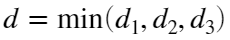A = [-1,0,-1.1];
B = [1,0,-1.1];
BmA = B-A;
den = BmA*BmA';
d3 = zeros(size(X));
for i = 1:numel(X)
P = [X(i),Y(i),Z(i)];
lamb = dot(P-A, BmA)/den;
lambt = min(1, max(0, lamb));
Q = A+lambt*BmA;
d3(i) = norm(P-Q)-0.2;
end
dd=min(d1,min(d2,d3));
dVal=0.2;
figure
patch(isosurface(X,Y,Z,dd,dVal,X),...
'FaceColor','interp','EdgeColor','none')
view(30,-15)
axis equal
grid
colormap gray
view([-51.53 19.80])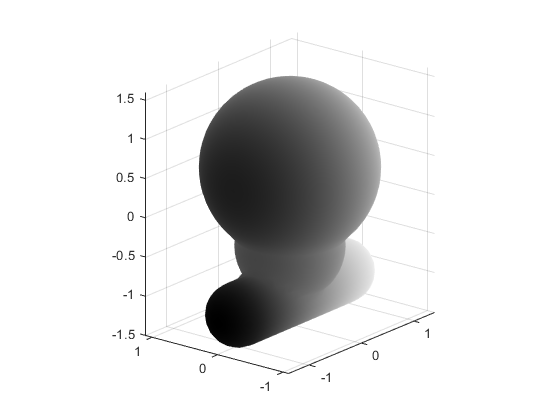Substractting two bodies:

You can also subtract two bodies if you consider the distance function% Add a new small sphere
dd=max(d1,-d2);
dVal=0.02;
figure
patch(isosurface(X,Y,Z,dd,dVal,X),...
'FaceColor','interp','EdgeColor','none')
view(30,-15)
axis equal
grid
colormap gray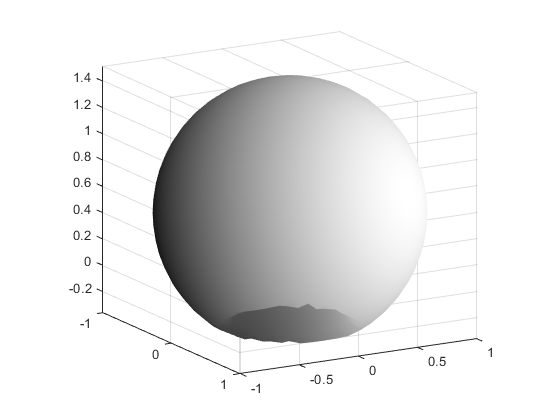%note: the boundary artifacts are due to the size of the discretization in
%the volume points (try a high resolution for improve).
If you want to have fun, I suggest you watch the video Painting a Selfie Girl, with Maths from Iñigo Quilez. It is an amazing application of these techniques.
© Numerical Factory 2021 (Agustí Roig in memoriam, who suggested me to implement this script for our students).